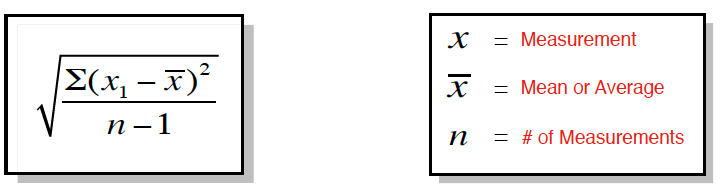Ch.1 - Intro to General ChemistryWorksheetSee all chapters
 Ch.1 - Intro to General Chemistry 2hrs & 53mins 0% complete Worksheet Ch.2 - Atoms & Elements 2hrs & 49mins 0% complete Worksheet Ch.3 - Chemical Reactions 3hrs & 25mins 0% complete Worksheet BONUS: Lab Techniques and Procedures 1hr & 38mins 0% complete Worksheet BONUS: Mathematical Operations and Functions 47mins 0% complete Worksheet Ch.4 - Chemical Quantities & Aqueous Reactions 3hrs & 30mins 0% complete Worksheet Ch.5 - Gases 3hrs & 47mins 0% complete Worksheet Ch.6 - Thermochemistry 2hrs & 28mins 0% complete Worksheet Ch.7 - Quantum Mechanics 2hrs & 35mins 0% complete Worksheet Ch.8 - Periodic Properties of the Elements 1hr & 57mins 0% complete Worksheet Ch.9 - Bonding & Molecular Structure 2hrs & 5mins 0% complete Worksheet Ch.10 - Molecular Shapes & Valence Bond Theory 1hr & 31mins 0% complete Worksheet Ch.11 - Liquids, Solids & Intermolecular Forces 3hrs & 40mins 0% complete Worksheet Ch.12 - Solutions 2hrs & 17mins 0% complete Worksheet Ch.13 - Chemical Kinetics 2hrs & 22mins 0% complete Worksheet Ch.14 - Chemical Equilibrium 2hrs & 26mins 0% complete Worksheet Ch.15 - Acid and Base Equilibrium 4hrs & 42mins 0% complete Worksheet Ch.16 - Aqueous Equilibrium 3hrs & 48mins 0% complete Worksheet Ch. 17 - Chemical Thermodynamics 1hr & 44mins 0% complete Worksheet Ch.18 - Electrochemistry 2hrs & 58mins 0% complete Worksheet Ch.19 - Nuclear Chemistry 1hr & 33mins 0% complete Worksheet Ch.20 - Organic Chemistry 3hrs 0% complete Worksheet Ch.22 - Chemistry of the Nonmetals 2hrs & 1min 0% complete Worksheet Ch.23 - Transition Metals and Coordination Compounds 1hr & 54mins 0% complete Worksheet

# Standard Deviation, Mean, Median & Mode

See all sections

Standard deviation measures how close our numerical data points are to one another and to the “true” value.

###### Mean Value Evaluation

Concept #1: Understanding Standard Deviation

Transcript

We're going to say that the standard deviation, so standard deviation, measures how close data results are to the mean or average value. We're going to say sometimes it's easy for us to look when we have a few numbers. We just simply look to see how close they are to one another, how close they are to the true value. But sometimes, we may require some help. That's the whole basis for standard deviation.
This is going to become an important idea when it comes to accuracy and precision in this chapter and also when you take your lab. Because when you're doing labs, labs are all based on how accurate and how precise can your measurements be when doing any of the experiments that you have to do.
We're going to say here, the equation for standard deviation is the square root, where we have the summation. This is sigma, the summation of x1 minus x squared divided by n minus one. We're going to say that x or x1 is simply a measurement. We're going to say that x with a bar on top of it is our mean or average of all the measurements added up and divided by the total number of measurements. This is our mean or average. Then we're going to say n, n represents the number of measurements that we have.
The equation might seem a little bit intimidating, but it's really easy as long as you can remember what it is and how do we plug in the numerical values that were given.Example #1: Calculate the standard deviation for the following results: 5.29, 5.35 and 5.31.

Practice: Calculate the standard deviation for the following results: 0.039, 0.061 and 5.3 x 10-2